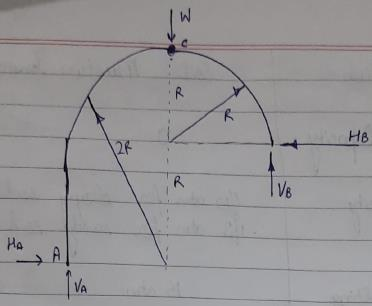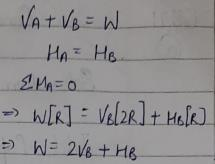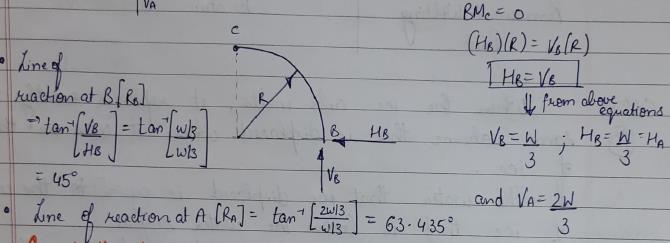Courses

# Test: Arches -1

## 10 Questions MCQ Test GATE Civil Engineering (CE) 2022 Mock Test Series | Test: Arches -1

Description
This mock test of Test: Arches -1 for GATE helps you for every GATE entrance exam. This contains 10 Multiple Choice Questions for GATE Test: Arches -1 (mcq) to study with solutions a complete question bank. The solved questions answers in this Test: Arches -1 quiz give you a good mix of easy questions and tough questions. GATE students definitely take this Test: Arches -1 exercise for a better result in the exam. You can find other Test: Arches -1 extra questions, long questions & short questions for GATE on EduRev as well by searching above.
QUESTION: 1

### In a two hinged arch an increase in temperature induces

Solution:

Increase in temperature in a two hinged arch (degree of indeterminacy one ) will cause horizontal thrust only.

Moment due to horizontal thrust is -Hy

So, max B.M will be at crown as crown has height value of y.

QUESTION: 2

Solution:
QUESTION: 3

### A three-hinged parabolic arch rib of span L and crown rise ' h ' carries a uniformly distributed superimposed load of intensity W per unit length. The hinges are located on two abutments at the same level and the third hinge at a quarter span location from left hand abutment. The horizontal thrust on the abutment is

Solution: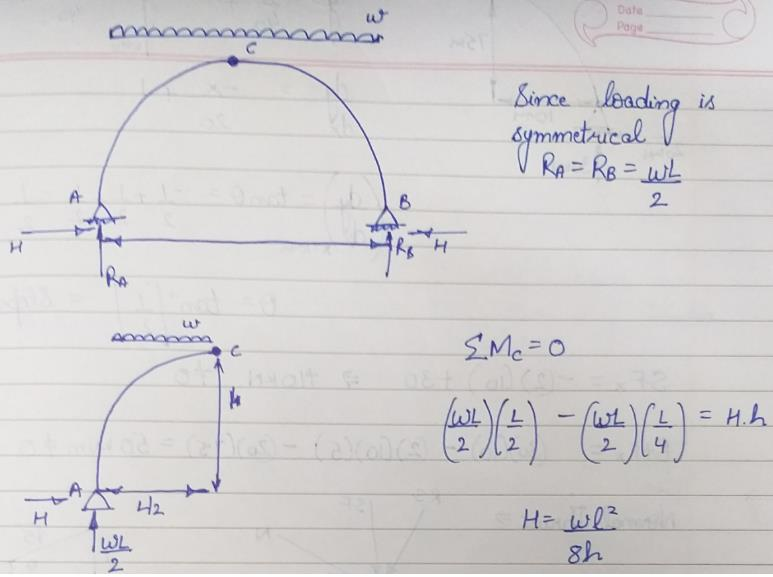QUESTION: 4

A symmetrical two-hinged parabolic arch rib has a span of 32 m between abutment pins at the same level and a central rise of 5 m. When a rolling load of 100 kN crosses the span, the maximum horizontal thrust at the hinges will be

Solution: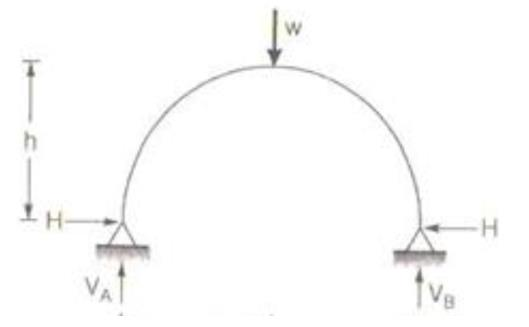Maximum Horizontal Thrust occurs when the load is at center and is given by
H = (25WL)/(128h)
W = Concentrated Load = 100kN
Span= L = 32m; Rise= h=5m
Substituting the values we get H=125kN

QUESTION: 5

A uniformly distributed load of 2 kN/m covers left half of the span of a three-hinged parabolic arch, span 40 m and central rise 10 m. Which of the following statements relating to different functions at the loaded quarter point are correct?
1. The slope is tan-1 (1/2)
2. The normal thrust is 6√5 kN.
3. The shear force is not zero
4. The bending moment is zero.

Select the correct answer using the codes' given below:

Solution: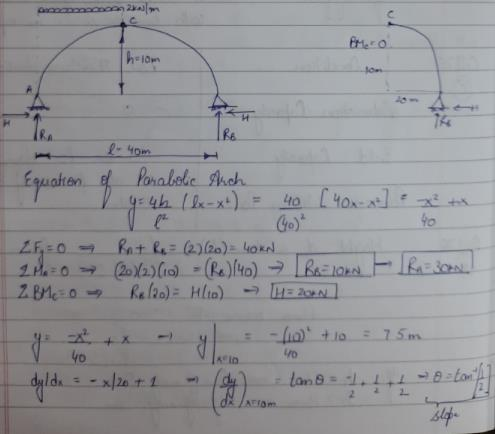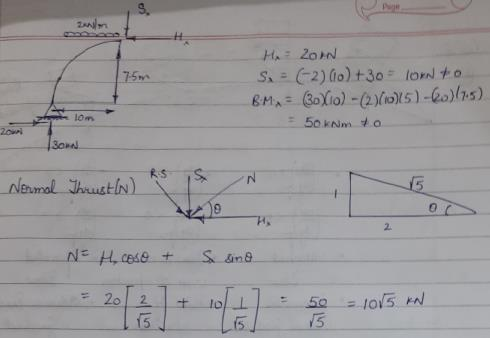QUESTION: 6

A three hinged arch is shown in the below figure. The influence line for the horizontal thrust is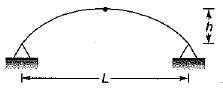Solution:
QUESTION: 7

For the two-hinged parabolic arch as shown in the below figure, which one of the following diagrams represents the shape of the bending moment variation.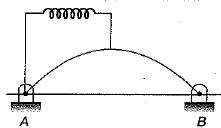Solution:
QUESTION: 8

A circular three-pinned arch of span 40 m and a rise of 8 m is hinged at the crown and springings. it carries a horizontal load of 100 kN per vertical metre on the left side. The horizontal thrust at the right springing will be

Solution:
QUESTION: 9

A symmetrical three-hinged parabolic arch of span L and rise h is hinged at springing and crown. It is subjected to a UDLw throughout the span. What is the bending moment at a section L/4 from the left support?

Solution:
QUESTION: 10

A three-hinged circular arch ACB is formed by two quadrants of circles AC and BC of radii 2R and R respectively with C as crown, as shown in the figure. Consider the following in respect of the reactive forces developed at supports A and B due to concentrated load at the crown: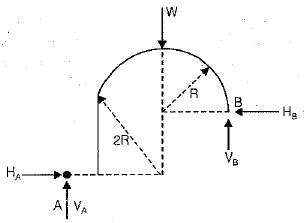1. Line of action of reaction RA at B is inclined at 45° to the horizontal.
2. H= HA = W/3
3. VB = 2VA
4. VA = VB + W/3

Which of the above is/are correct?

Solution: